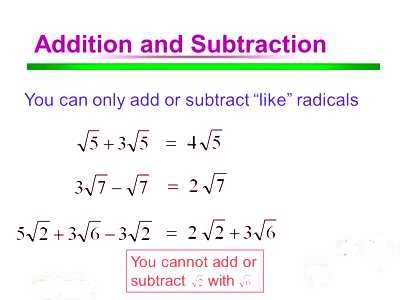A root of a positive real quantity is called a surd if its value cannot be exactly determined. It is a number that can’t be simplified to remove a square root (or cube root etc). For example, each of the quantities √3, ∛7, ∜19, (16)^2/5 etc. is a surd.

More Examples:

• √2 (square root of 2) can’t be simplified further so it is a surd
• √4 (square root of 4) CAN be simplified to 2, so it is NOT a surd

In addition and subtraction of surds, we will learn how to find the sum or difference of two or more surds only when they are in the simplest form of like surds.

Follow the following steps to find the addition and subtraction of two or more surds.

Step I: Convert each surd in its simplest mixed form.

Step II: Then find the sum or difference of rational co-efficient of like surds.

Step III: Finally, to get the required sum or difference of like surds multiply the result obtained in step II by the surd-factor of like surds.

Step IV: The sum or difference of unlike surds is expressed in a number of terms by connecting them with a positive sign (+) or negative (-) sign.

Adding and subtracting surds are simple- however, we need the numbers being square rooted (or cube rooted etc) to be the same.

4√7 – 2√7 = 2√7.

5√2 + 8√2 = 13√2

Note: 5√2 + 3√3 cannot be manipulated because the surds are different (one is √2 and one is √3).

However, if the number in the square root sign isn’t prime, we might be able to split it up in order to simplify an expression.Examples of Addition and Subtraction of Surds:

Find the sum of √12 and √27.

Solution:

Sum of √12 and √27

= √12 + √27

Step I: Express each surd in its simplest mixed form;

= 2√(2⋅2⋅3) + √(3⋅3⋅3)

= 2√3 + 3√3

Step II: Then find the sum of rational co-efficient of like surds.

= 5√3

Subtract 2√45 from 4√20.

Solution:

Subtract 2√45 from 4√20

= 4√20 – 2√45

Now convert each surd to its simplest form

= 4√(2⋅2⋅5) – 2√(3⋅3⋅5)

= 8√5 – 6√5

Clearly, we see that 8√5 and 6√5 are like surds.

Now find the difference of rational co-efficient of like surds

= 2√5.

Information Source: